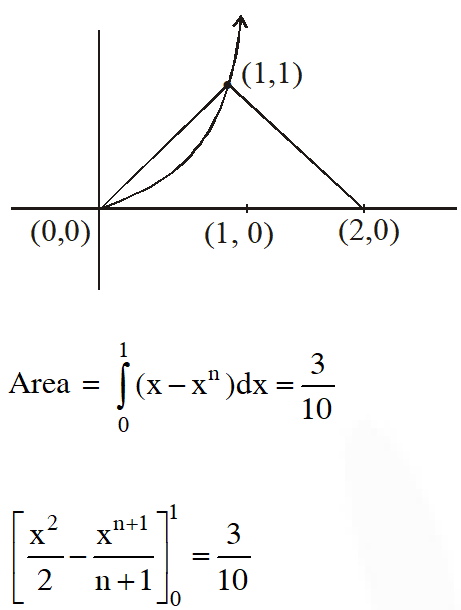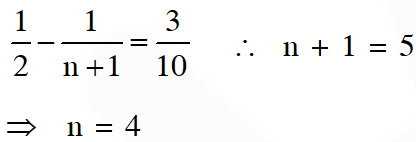Most Affordable JEE | NEET | 8,9,10 Preparation by Kota's Top IITian Doctor Faculties

# Area Under The Curve - JEE Advanced Previous Year Questions with SolutionsJEE Advanced Previous Year Questions of Math with Solutions are available at eSaral. Practicing JEE Advanced Previous Year Papers Questions of mathematics will help the JEE aspirants in realizing the question pattern as well as help in analyzing weak & strong areas. eSaral helps the students in clearing and understanding each topic in a better way. eSaral also provides complete chapter-wise notes of Class 11th and 12th both for all subjects. Besides this, eSaral also offers NCERT Solutions, Previous year questions for JEE Main and Advance, Practice questions, Test Series for JEE Main, JEE Advanced and NEET, Important questions of Physics, Chemistry, Math, and Biology and many more. Download eSaral app for free study material and video tutorials.
Q. Area of the region bounded by the curve $y=e^{x}$ and $\operatorname{lines} x=0$ and $y=e$ is (A) e – 1 (B) $\int_{1}^{\mathrm{e}} l \mathrm{n}(\mathrm{e}+1-\mathrm{y}) \mathrm{d} \mathrm{y}$ (C) $\mathrm{e}-\int_{0}^{1} \mathrm{e}^{\mathrm{x}} \mathrm{d} \mathrm{x}$ (D) $\int_{1}^{\mathrm{e}} \ln \mathrm{y} \mathrm{d} \mathrm{y}$ [JEE 2009, 4]
Ans. (B,C,D) $\mathrm{A}=\int_{1}^{e} \ln y \mathrm{d} y$ Apply $=\int_{1}^{e} \ln (e+1-y) d y$ $\mathrm{Also}, \mathrm{A}=\operatorname{ar}(\mathrm{O} \mathrm{ABC})-\operatorname{ar}(\mathrm{OABD})$ $=\mathrm{e}-\int_{1}^{e} \mathrm{e}^{\mathrm{x}} \mathrm{d} \mathrm{x}$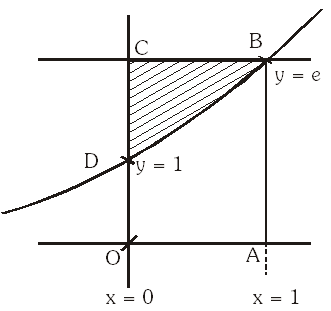Q. Comprehension (3 questions together) : Consider the poly nomial $\mathrm{f}(\mathrm{x})=1+2 \mathrm{x}+3 \mathrm{x}^{2}+4 \mathrm{x}^{3} .$ Let $\mathrm{s}$ be the sum of all distinct real roots of $\mathrm{f}(\mathrm{x})$ and let $\mathrm{t}=|\mathrm{s}| .$ (i) The real number s lies in the interval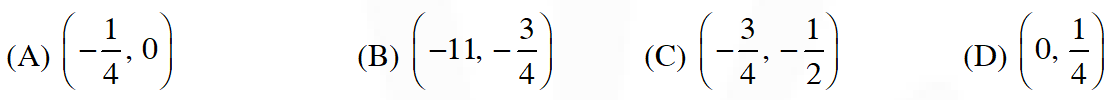(ii) The area bounded by the curve y = f(x) and the lines x = 0, y = 0 and x = t, lies in the interval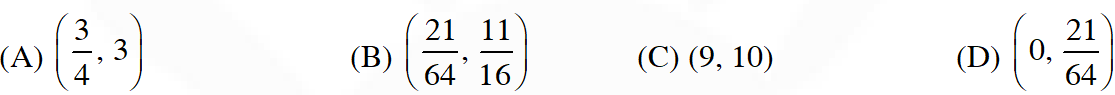(iii) The function f'(x) is (A) increasing in $\left(-\mathrm{t},-\frac{1}{4}\right)$ and decreasing in $\left(-\frac{1}{4}, \mathrm{t}\right)$ (B) decreasing in $\left(-\mathrm{t},-\frac{1}{4}\right)$ and increasing in $\left(-\frac{1}{4}, \mathrm{t}\right)$ (C) increasing in $(-\mathrm{t}, \mathrm{t})$ (D) decreasing in $(-\mathrm{t}, \mathrm{t})$ [JEE 2010, 3+3+3]
Ans. ((i) $\mathrm{C} \quad$ (ii) $\mathrm{A} \quad$ (iii) $\mathrm{B}$)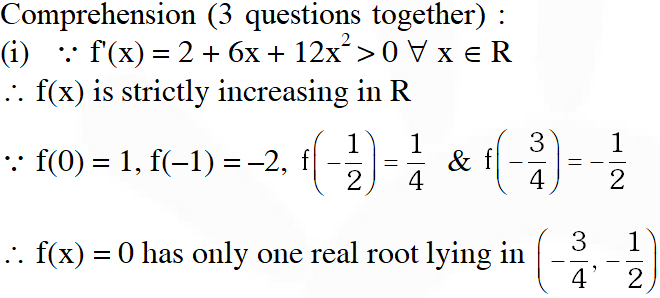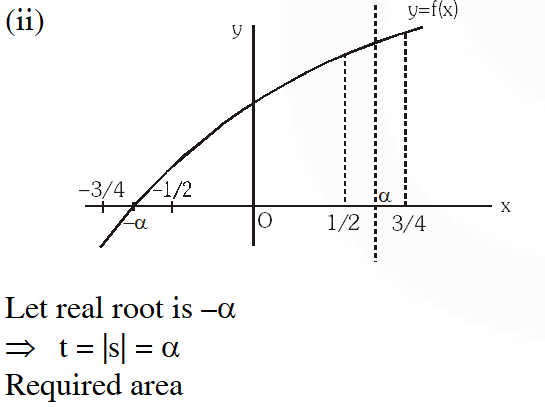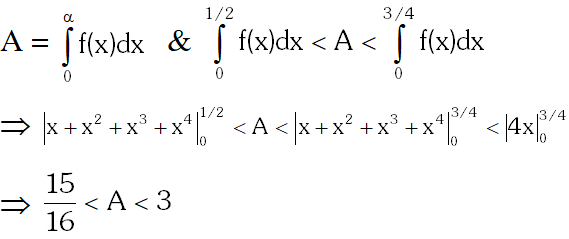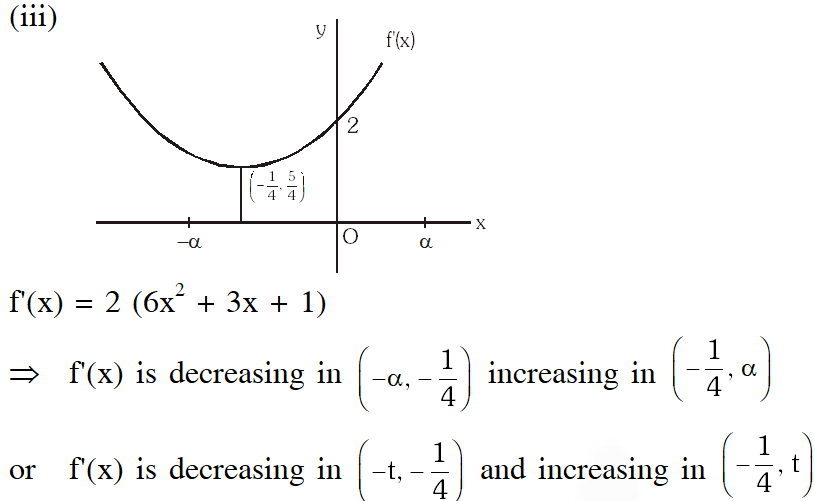Q. (A) Let the straight line $\mathrm{x}=\mathrm{b}$ divide the area enclosed by $\mathrm{y}=(1-\mathrm{x})^{2}, \mathrm{y}=0$ and $\mathrm{x}=0$ into two parts $\mathrm{R}_{1}(0 \leq \mathrm{x} \leq \mathrm{b}) \mathrm{d}$ and $\mathrm{R}_{2}(\mathrm{b} \leq \mathrm{x} \leq 1)$ such that $\mathrm{R}_{1}-\mathrm{R}_{2}=\frac{1}{4} .$ Then $\mathrm{b}$ equals (A) $\frac{3}{4}$ (B) $\frac{1}{2}$ (C) $\frac{1}{3}$ (D) $\frac{1}{4}$ (B) Let $f:[-1,2] \rightarrow[0, \infty)$ be a continuous function such that $f(\mathrm{x})=f(1-\mathrm{x})$ for all $\mathrm{x} \in[-1,2]$. Let $\mathrm{R}_{1}=\int_{-1}^{2} \mathrm{x} f(\mathrm{x}) \mathrm{d} \mathrm{x}$, and $\mathrm{R}_{2}$ be the area of the region bounded by $\mathrm{y}=f(\mathrm{x}), \mathrm{x}=-1, \mathrm{x}=2$,and the x-axis. Then- (A) $\mathrm{R}_{1}=2 \mathrm{R}_{2}$ (B) $\mathrm{R}_{1}=3 \mathrm{R}_{2}$ (C) $2 \mathrm{R}_{1}=\mathrm{R}_{2}$ (D) $3 \mathrm{R}_{1}=\mathrm{R}_{2}$ [JEE 2011, 3+3]
Ans. ((A) B (B) C )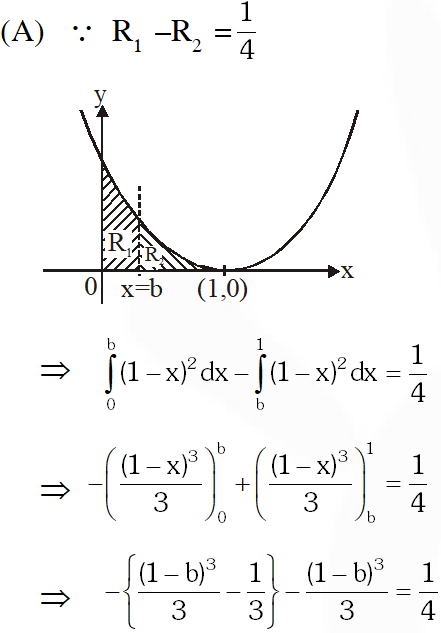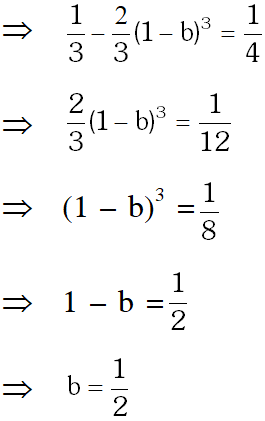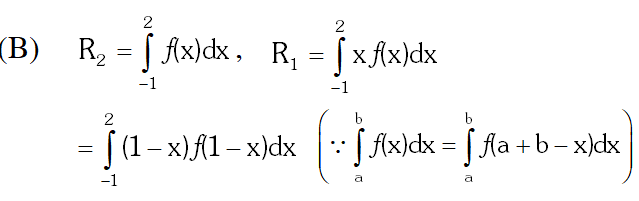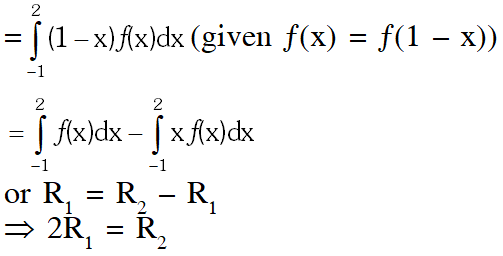Q. The area enclosed by the curve $y=\sin x+\cos x$ and $y=|\cos x-\sin x|$ over the interval $\left[0, \frac{\pi}{2}\right]$ is (A) $4(\sqrt{2}-1)$ (B) $2 \sqrt{2}(\sqrt{2}-1)$ (C) $2(\sqrt{2}+1)$ (D) $2 \sqrt{2}(\sqrt{2}+1)$ [JEE(Advanced) 2013, 2M]
Ans. (B)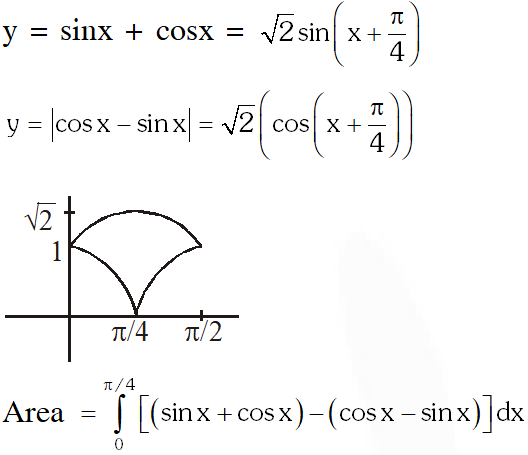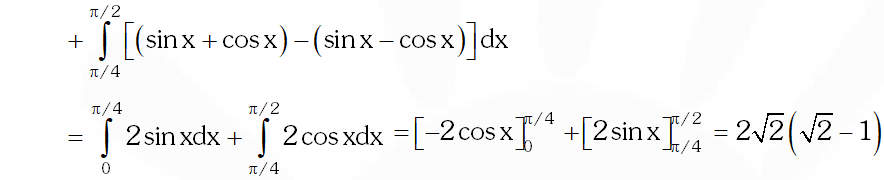Q. Let $\mathrm{F}(\mathrm{x})$$=\int_{x}^{x^{2}+\frac{\pi}{6}}$ $2 \cos ^{2}$ tdt for all $\mathrm{x} \in \mathbb{U}$ and $f:\left[0, \frac{1}{2}\right] \rightarrow[0, \infty)$ be a continuous function. For $\mathrm{a} \in\left[0, \frac{1}{2}\right],$ if $\mathrm{F}^{\prime}(\mathrm{a})+2$ is the area of the region bounded by x = 0, y = 0, y = ƒ(x) and x = a, then ƒ(0) is [JEE 2015, 4M, –0M]
Ans. 3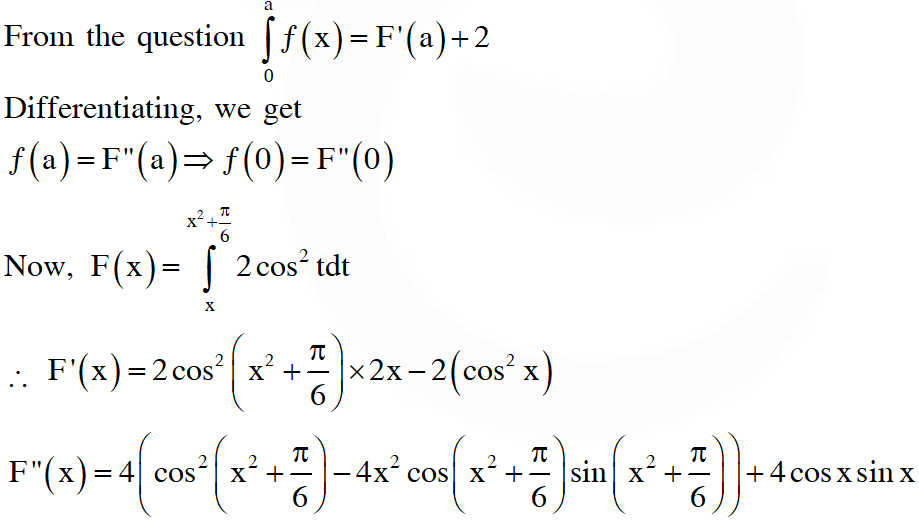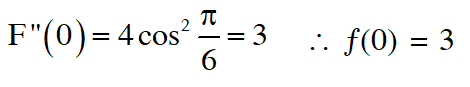Q. Area of the region $\{ \left(\mathrm{x}, \mathrm{y}) \in \mathbb{D}^{2}: \mathrm{y} \geq \sqrt{|\mathrm{x}+3|, 5 \mathrm{y} \leq \mathrm{x}+9 \leq 15\}}$ is equal to - \right. (A) $\frac{1}{6}$ (B) $\frac{4}{3}$ (C) $\frac{3}{2}$ (D) $\frac{5}{3}$ [JEE(Advanced) 2016]
Ans. (C)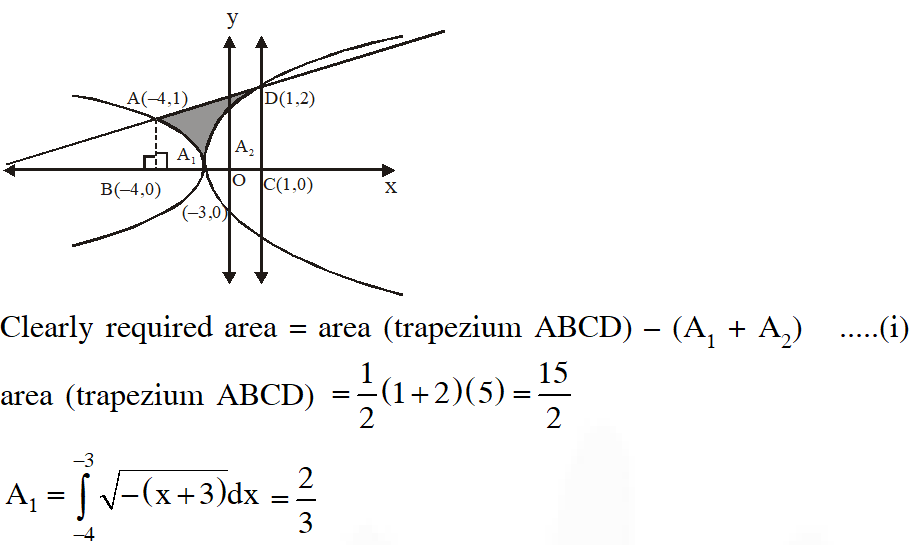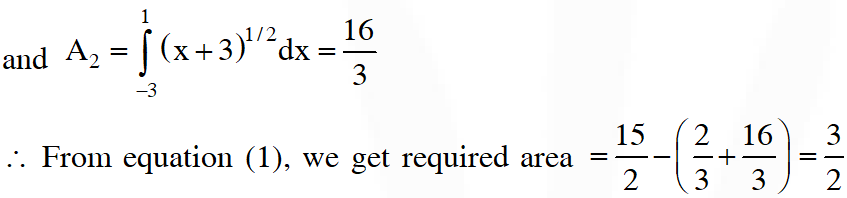Q. If the line $x=\alpha$ divides the area of region $R=\left\{(x, y) \in \square^{2}: x^{3} \leq y \leq x, 0 \leq x \leq 1\right\}$ into two equal parts, then (A) $\frac{1}{2}<\alpha<1$ (B) $\alpha^{4}+4 \alpha^{2}-1=0$ (C) $0<\alpha \leq \frac{1}{2}$ (D) $2 \alpha^{4}-4 \alpha^{2}+1=0$ [JEE(Advanced) 2017]
Ans. (A,D)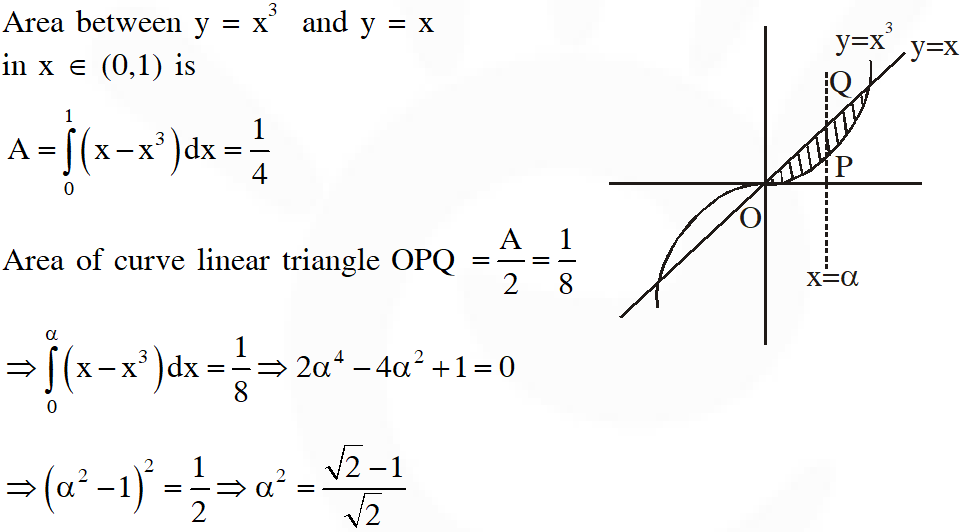Q. Let $f:[0, \infty) \rightarrow \square$ be a continuous function such that $f(\mathrm{x})=1-2 \mathrm{x}+\int_{0}^{\mathrm{x}} \mathrm{e}^{\mathrm{x}-\mathrm{t}} f(\mathrm{t}) \mathrm{d} \mathrm{t}$ for all $\mathrm{x} \in[0, \infty) .$ Then, which of the following statement(s) is (are) TRUE? (A) The curve y = ƒ(x) passes through the point (1, 2) (B) The curve y = ƒ(x) passes through the point (2, –1) (C) The area of the region $\{(x, y) \in[0,1] \times \square: f(x) \leq y \leq \sqrt{1-x^{2}}\}$ is $\frac{\pi-2}{4}$ (D) The area of the region $\{(x, y) \in[0,1] \times \square: f(x) \leq y \leq \sqrt{1-x^{2}}\}$ is $\frac{\pi-1}{4}$ [JEE(Advanced) 2018]
Ans. (B,C)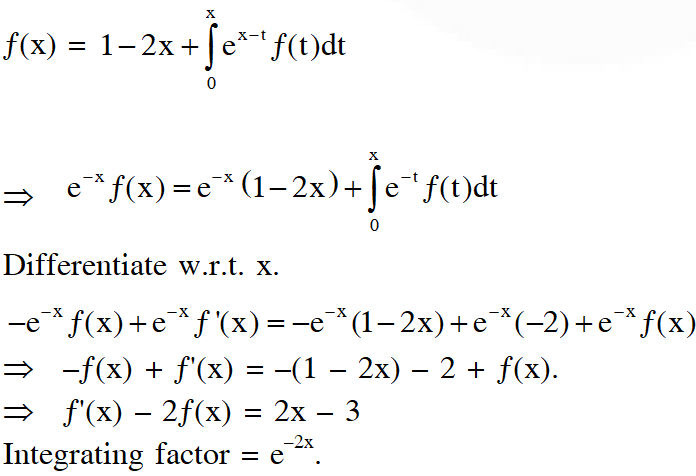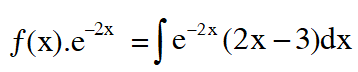Q. A farmer $F_{1}$ has a land in the shape of a triangle with vertices at $P(0,0), Q(1,1)$ and $R(2,)$ 0 . From this land, a neighbouring farmer $F_{2}$ takes away the region which lies between the side $P Q$ and a curve of the form $y=x^{n}(n>1) .$ If the area of the region taken away by the farmer $F_{2}$ is exactly $30 \%$ of the area of $\triangle P Q R,$ then the value of $n$ is
Ans. 4